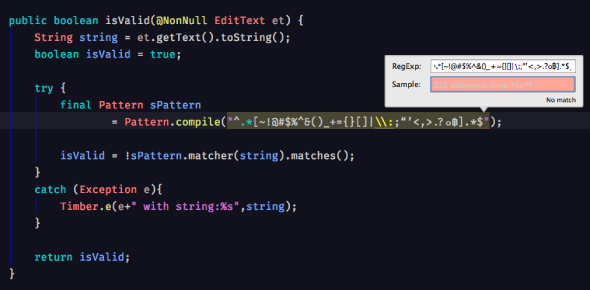# Java Regex Quiz

10 Questions | Total Attempts: 37SettingsHow much do you know about Java Regex? In Java, Regex, also known as Regular Expression is an API that helps in defining a pattern that can be used to search, edit or manipulate a string. Take the Java Regex quiz with a set of 10 multiple choice questions and answers to test your knowledge. To get further information, you can also check the Java Regex online course.

• 1.
Which class of objects is utilized to compile regular expressions?
• A.

Matcher class

• B.

Pattern class

• C.

String class

• D.

None of the preceding

• 2.
What does \d mean?
• A.

Range of numbers

• B.

Range of alphabets

• C.

Range of special characters

• D.

None of the preceding

• 3.
Which of the following regular expressions in Java matches the string's end?
• A.

\d

• B.

/d

• C.

/z

• D.

\z

• 4.
Which of the following is correct?
• A.

A string regular expression must first be compiled into an instance of the Pattern class.

• B.

A string regular expression can be compiled into an instance of the Pattern class.

• C.

A string regular expression must first be compiled into an instance of the Matcher class.

• D.

A string regular expression can be compiled into an instance of the Pattern class.

• 5.
Which of the following can be used to match arbitrary number of characters?
• A.

Kleen Star

• B.

Kleen Plus

• C.

Both a & b

• D.

None

• 6.
A whitespace special character \s will match which of the following white spaces?
• A.

The tab

• B.

The new line

• C.

The carriage return

• D.

All of the preceding

• 7.
Which of the following about n+ is correct?
• A.

Matches a string that contains zero n only

• B.

Matches a string that contains one n only

• C.

Matches a string that contains at least one n

• D.

Matches a string that contains more than one n

• 8.
Which of the following statements is correct?
• A.

A regular expression can only be of one character

• B.

A regular expression can only be a complicated pattern

• C.

A regular expression can be a single expression as well as complicated pattern

• D.

None

• 9.
MatchResult is known as:
• A.

Regex class

• B.

Regex library

• C.

Regex engine

• D.

All of the preceding

• 10.
The dot is used to represent:
• A.

A string

• B.

An integer

• C.

A single character

• D.

Multiple characters

Related TopicsBack to top